# Increment Cell Reference – Excel & Google Sheets

This tutorial will demonstrate how to increment cell references by using the OFFSET Function in Excel and Google Sheets.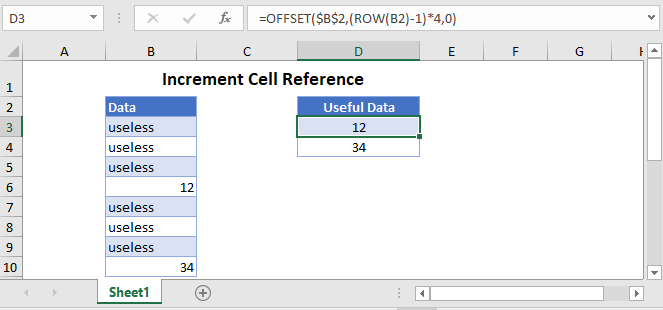## Increment Cell Reference

When copying formulas across rows or columns, if your cell references are not locked, they will move proportionally. Additionally, if you insert rows or columns, your cell reference will refer to the original row or column. Not the new adjacent row / column.

If you want to increment cell references with a foolproof method you can use the OFFSET Function.

### Increment Cell Reference in a Column

First, let’s look at an example. This example will increment cell references by 4 rows at a time using the OFFSET and ROW Functions:

``=OFFSET(\$B\$2,(ROW(B2)-1)*4,0)``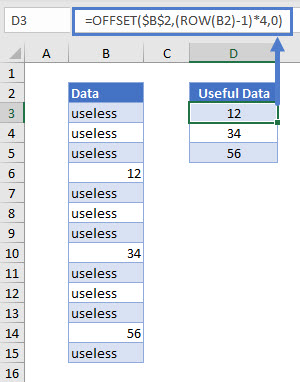Let’s see how this formula works exactly.

#### ROW Function

If you only have useful data in every nth row, you need to increase the cell reference by n. We can use the ROW Function to find the relevant row numbers.

``=(ROW(B2)-1)*4``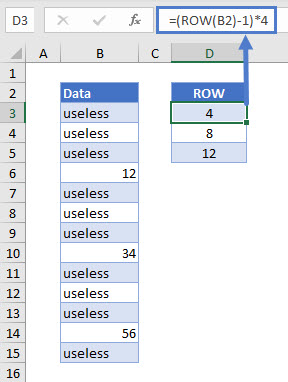In the example above, we only need data from every 4th row (n = 4). Starting at the header of your dataset (here, cell B2), use the formula above to find the row numbers for every 4th row.

#### OFFSET Function

The OFFSET Function returns the value of the cell that is a specified number of rows and columns from the given cell.

``=OFFSET(\$B\$2,D3,0)``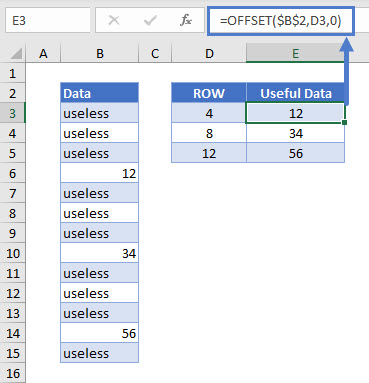Here, we start at the dataset header (cell B2) and increase the row by 4 (cell D3). Since we are staying in the same column, the cols argument of this function is set to 0. The \$s in “\$B\$2” lock the cell reference, so that cell E3 also starts at the data header when calculating the offset.

Combining these functions gives us our original formula:

``=OFFSET(\$B\$2,(ROW(B2)-1)*4,0)``

### Increment Cell Reference in a Row

If you want to increase cell references by more than 1 when filling formulas across a row, you can combine the OFFSET Function with the COLUMN Function.

``=OFFSET(\$B\$2,0,(COLUMN(B2)-1)*4)``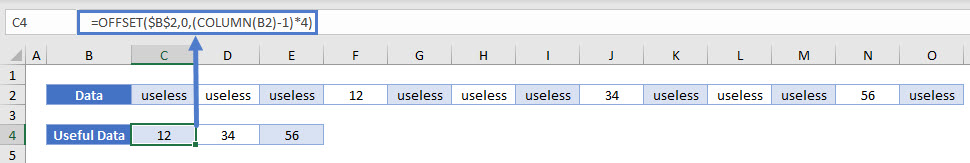This formula works exactly the same way as the above one for incrementing cell reference in a column. The only difference is that the rows argument of the function is set to zero and the cols argument is incremented by 4.

## Increment Cell Reference in Google Sheets

These formulas work exactly the same in Google Sheets as in Excel.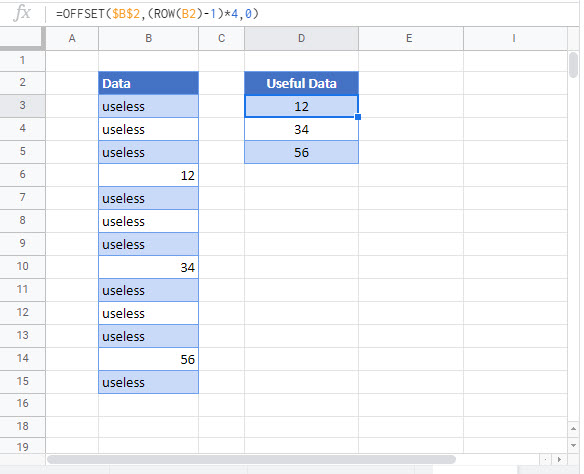### Excel Practice Worksheet

Practice Excel functions and formulas with our 100% free practice worksheets!